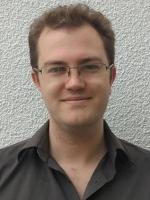Faculty of MathematicsProfessor of Mathematics

Research Interests: Algebraic and Geometric Topology, in particular: mapping class groups, moduli spaces, cobordism categories, spaces of manifolds, surgery, configuration spaces, characteristic classes, K-theory and applications of homotopy theory to geometry.

Publications

An ${\bf H}P^2$-bundle over $S^4$ with nontrivial $\hat{A}$-genus
M Krannich, A Kupers, O Randal-Williams
– Comptes Rendus. Mathématique
(2021)
359,
149
Tautological rings and stabilisation
O Randal-Williams
– Glasgow Mathematical Journal
(2021)
64,
231
A vanishing theorem for tautological classes of aspherical manifolds
F Hebestreit, M Land, W Lück, O Randal-Williams
– Geometry & Topology
(2021)
25,
47
Framings of $W_{g,1}$
A Kupers, O Randal-Williams
– The Quarterly Journal of Mathematics
(2021)
72,
1029
Mapping class groups of simply connected high-dimensional manifolds need not be arithmetic
M Krannich, O Randal-Williams
– Comptes Rendus Mathématique
(2020)
358,
469
On the cohomology of Torelli groups
A Kupers, O Randal-Williams
– Forum of Mathematics, Pi
(2020)
8,
e7
The cohomology of Torelli groups is algebraic
A Kupers, O Randal-Williams
– Forum of Mathematics Sigma
(2020)
8,
e64
Homology of hurwitz spaces and the cohen-lenstra heuristic for function fields: [after Ellenberg, Venkatesh, and Westerland]
O Randal-Williams
– Astérisque
(2020)
422,
469
Semi-simplicial spaces
J Ebert, O Randal-Williams
– Algebraic & Geometric Topology
(2019)
19,
2099
$E_{2}$-cells and mapping class groups
S Galatius, A Kupers, O Randal-Williams
– Publications Mathématiques de l'Institut des Hautes Études Scientifiques
(2019)
130,
1
• 1 of 6
• >

Research Group

Algebraic Geometry

E2.06

01223 764291